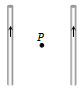• Subject:

...

• Topic:

...

Two long straight wires are set parallel to each other. Each carries a current i in the same direction and the separation between them is 2r. The intensity of the magnetic field midway between them is(a) ${\mu }_{0}i/r$                               (c) Zero

(b) $4{\mu }_{0i/r}$                              (d) ${\mu }_{0}i/4r$